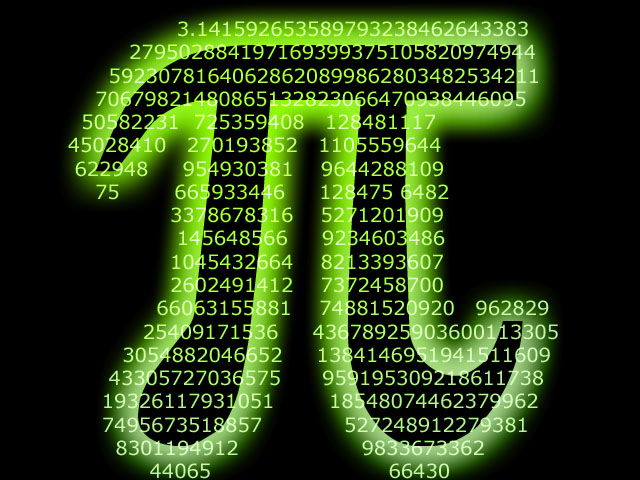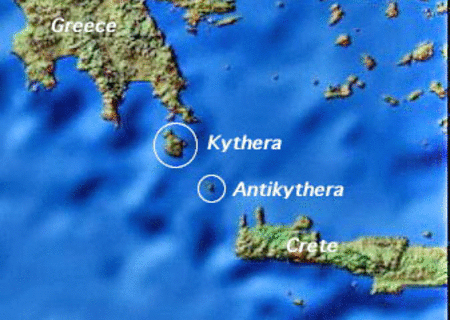# Fallen from Outer Space…# Record-Breaking Space Dive

14th October 2012.  During his ultimate high-altitude parachute jump, Felix Baumgartner spends approximately 4 minutes and 22 seconds in freefall, at the maximum speed of 1,342 kilometres per hour.  Continue reading Fallen from Outer Space…

#Fiat Lux…

Light.  Most of us take it for granted during the day.  And at night, we have learned to domesticate it.  Light, the natural agent that stimulates our sense of sight and makes things around us visible.  Continue reading Let There Be Light…

#Physical Constants (SI Units)

Avogadro Constant$N_{A} = 6.022 \times 10^{23} mol^{-1}$

Bohr Radius$a_0 = 5.29 \times 10^{-11} m$

Boltzmann Constant$k = 1.381 \times 10^{-23} JK^{-1}$

Charge on an Electron$e = - 1.602 \times 10^{-19} C$

Gravitational Constant$G = 6.673 \times 10^{-11} Nm^{2} kg^{-2}$

Magnitude of Acceleration due to Gravity$g = 9.81 m s^{-2}$

Permeability of Free Space$\mu_{0} = 4 \pi \times 10^{-7} T m A^{-1}$

Permittivity of Free Space$\epsilon_{0} = 8.854 \times 10^{-12} C^{2} N^{-1} m^{-2}$

Speed of Light in a vacuum$c = 2.998 \times 10^{8} m s^{-1}$

#Astronomical Beginnings

Early astronomers already make the distinction between stars and planets, as the former remain relatively fixed for centuries, while the latter wander an appreciable amount in a comparatively short time.  But that’s not all!

## Little 'Bytes' about Natural Phenomena, Theoretical Physics and the Latest Worldwide Scientific Findings. Edited from Glasgow, Scotland.